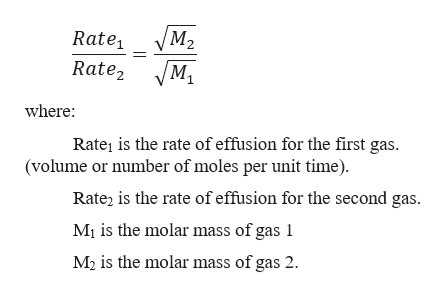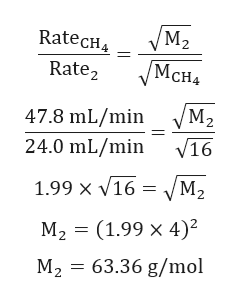# The rate of a particular gas was measured and found to be 24.0 mL/min. Under the same conditions, the rate of effusion of pure methane (CH4) gas is 47.8 mL/min. What is the molar mass of the unknown gas?

Question
1 views

The rate of a particular gas was measured and found to be 24.0 mL/min. Under the same conditions, the rate of effusion of pure methane (CH4) gas is 47.8 mL/min. What is the molar mass of the unknown gas?

check_circle

Step 1

Graham`s law of diffusion: the rate of effusion of a gas is inversely proportional to the square root of the molar mass of its particles.

Step 2

For two gasses:help_outlineImage TranscriptioncloseM2 Rate M1 Rate2 where: Rate1 is the rate of effusion for the first gas. (volume or number of moles per unit time) Rate2 is the rate of effusion for the second gas. M1 is the molar mass of gas 1 M2 is the molar mass of gas 2. fullscreen
Step 3

Calculation for molar...help_outlineImage TranscriptioncloseМ2 RateCH МсHA Rate2 M2 47.8 mL/min V16 24.0 mL/min 1.99 x v16 = М, %3D (1.99 х 4)? M2 63.36 g/mol fullscreen

### Want to see the full answer?

See Solution

#### Want to see this answer and more?

Solutions are written by subject experts who are available 24/7. Questions are typically answered within 1 hour.*

See Solution
*Response times may vary by subject and question.
Tagged in

### Chemistry